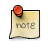Navigation C API Pages Python bindings Applications

## Event Queue

Event queue is a queue to store input events.This API is semi internal, normally the queue is used indirectly by the backend code.

### Event Queue API

```#include <gfxprim.h>
/* or */
#include <input/gp_ev_queue.h>

/*
* Initializes event queue passed as a pointer. The events array must be
* queue_size long.
*
* If queue_size is set to zero, default value is expected.
*/
void gp_ev_queue_init(gp_ev_queue *self,
unsigned int screen_w, unsigned int screen_h,
unsigned int queue_size, int flags);```

The initialization functions takes pointer to a memory large enough to hold an event queue structure and array of queue_size events.

```#include <gfxprim.h>
/* or */
#include <input/gp_ev_queue.h>

unsigned int gp_ev_queue_events(gp_ev_queue *self);```

Returns number of queued events.

```#include <gfxprim.h>
/* or */
#include <input/gp_ev_queue.h>

gp_event *gp_ev_queue_get(gp_ev_queue *self);```

In case there are any events queued a pointer to a top event in the queue is returned. The pointer is valid until next call to `gp_ev_queue_get()`.

The pointer is also invalidated by a call to `gp_ev_queue_put_back()`.

If there are no events queued the call returns NULL.

```#include <gfxprim.h>
/* or */
#include <input/gp_ev_queue.h>

gp_event *gp_ev_queue_peek(gp_ev_queue *self);```

Same as `gp_ev_queue_get()` but the top event is not removed from the queue.

```#include <gfxprim.h>
/* or */
#include <input/gp_ev_queue.h>

void gp_ev_queue_put_back(gp_ev_queue *self, gp_event *ev);```

Puts event to the top of the queue. Useful for putting back event that has been removed from the queue.

```#include <gfxprim.h>
/* or */
#include <input/gp_ev_queue.h>

/*
* Inject event that moves cursor by rx and ry.
*
* If timeval is NULL, current time is used.
*/
void gp_ev_queue_push_rel(gp_ev_queue *self,
int32_t rx, int32_t ry, struct timeval *time);

/*
* Produces relative event that moves cursor to the point x, y.
*
* If timeval is NULL, current time is used.
*/
void gp_ev_queue_push_rel_to(gp_ev_queue *self,
uint32_t x, uint32_t y, struct timeval *time);

/*
* Inject absolute event.
*
* If timeval is NULL, current time is used.
*/
void gp_ev_queue_push_abs(gp_ev_queue *self,
uint32_t x, uint32_t y, uint32_t pressure,
uint32_t x_max, uint32_t y_max, uint32_t pressure_max,
struct timeval *time);

/*
* Inject event that changes key state (i.e. press, release, repeat).
*
* If timeval is NULL, current time is used.
*/
void gp_ev_queue_push_key(gp_ev_queue *self,
uint32_t key, uint8_t code, struct timeval *time);

/*
* Inject window resize event
*/
void gp_ev_queue_push_resize(gp_ev_queue *self,
uint32_t w, uint32_t h, struct timeval *time);

/*
* Inject common event.
*/
void gp_ev_queue_push(gp_ev_queue *self,
uint16_t type, uint32_t code, int32_t value,
struct timeval *time);
```

These functions are used for puting events into the event queue. If pointer to the timeval structure is NULL the event time will be filled with exact time the event was added to the queue.